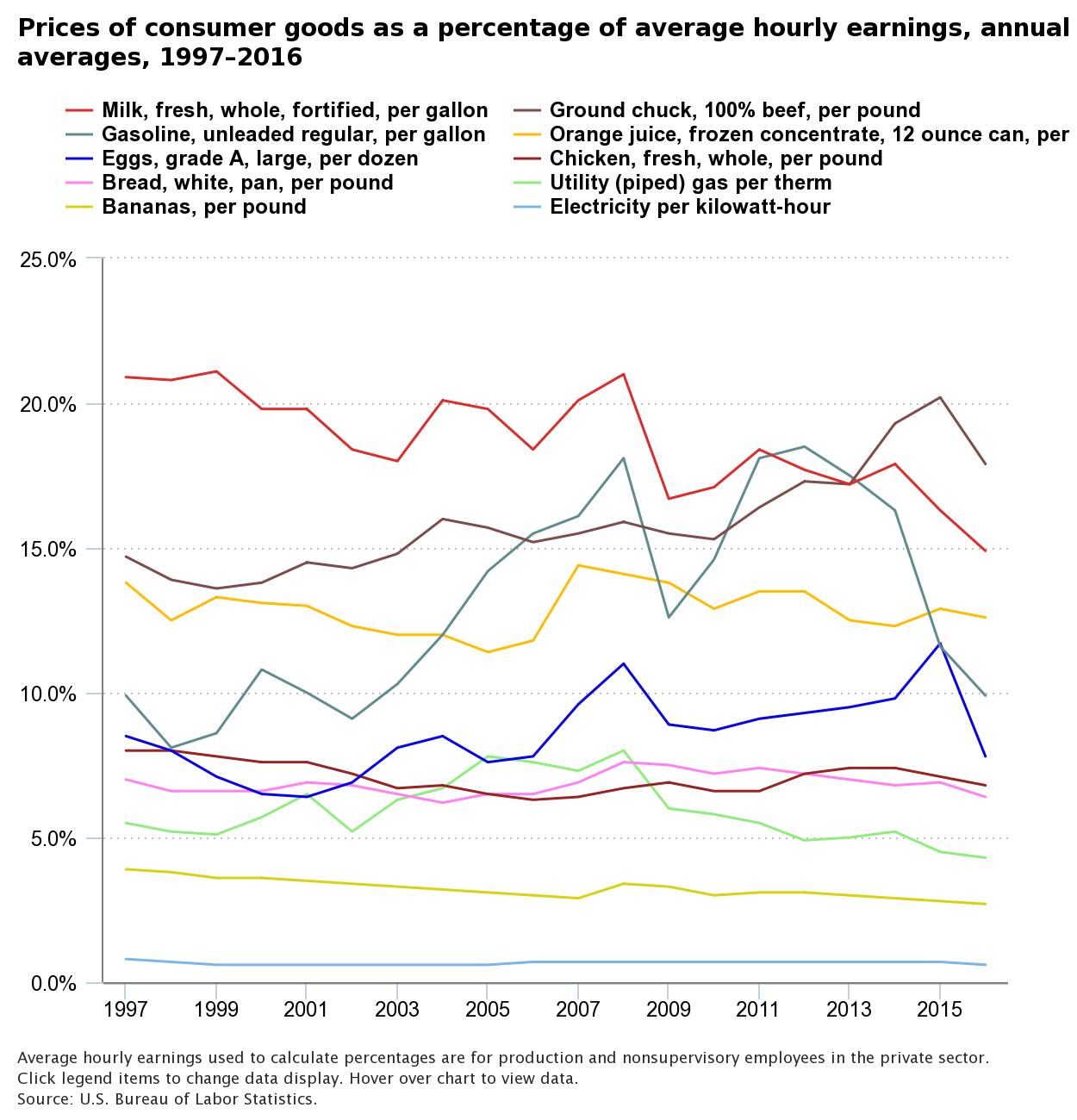## The Difference Between Nominal and Real Measurements

### Learning Objectives

• Contrast nominal measurements and real measurements of economic statistics

## Nominal Measurements and Real Measurements

When examining economic statistics, there is a crucial distinction you need to be aware of. The distinction is between nominal and real measurements, which refers to whether or not the measurement has been corrected for inflation. This is important because inflation distorts economic magnitudes, making them look bigger than they really are.Figure 1. If basketball superstar Michael Jordan made 78.3 million dollars in 1997, but soccer phenomenon Christiano Ronaldo made 93 million dollars in 2017, who made more? In order to find out, you need to adjust for inflation! (The answer is Jordan).

Looking at economic statistics without considering inflation is like looking through a pair of binoculars and trying to guess how close something is: unless you know how strong the lenses are, you cannot guess the distance very accurately. Take GDP for example. If you do not know the inflation rate, it is difficult to figure out if a rise in GDP is due mainly to a rise in the overall level of prices or to a rise in quantities of goods produced.

The nominal value of any economic statistic means that we measure the statistic in terms of actual prices that exist at the time. For example, nominal GDP in 2015 is measured as the quantity of each final good and service produced in 2015 times the price at which it was sold in 2015. Similarly, nominal GDP in 2016 is measured using 2016 prices. (If you’re thinking “What else would it be,” be patient.) If you look at a table or graph of economic data and the label says “billions of dollars,” you can be sure that you’re looking at nominal data.

The real value refers to the same statistic after it has been adjusted for inflation. We will explain the details of how this is done shortly, but for now just know that real values are computed using prices that existed in a single year, say 2015. In other words, real GDP in 2016 is measured as the quantity of each final good and service produced in 2016 times the price which existed in 2015. For this reason, real data are sometimes described as “constant dollars” or “2015 dollars.” Thus, if you look at a table or graph of economic data and the label says “billions of 2015 dollars,” you know that you’re looking at real data.

Another way to explore the real value of a good is to compare the prices of goods as percentages of hourly wages across time, as shown in Figure 2. Because this measurement is independent from prices and therefore inflation, it estimates the real cost of goods and services.Figure 2. The Real Cost of Consumer Items. This graph shows how the price of ground chuck beef would cost an hourly worker 17.9% of their hourly wage (11 minutes of work) in 2016. A gallon of whole milk would cost 9 minutes of work, and a gallon of gas would cost 6 minutes.

Here’s the thing: most data are collected in nominal terms, but generally it is real measurements that are more important. Let’s read on to look at the details of how we convert from one to the other.

### Watch It

Watch this video to review the differences and the need for differentiating between real and nominal GDP.

### Glossary

nominal value:
an economic statistic measured using actual market prices; i.e. nominal values are not adjusted for inflation; contrast with real value
real value:
an economic statistic measured after it has been adjusted for inflation; contrast with nominal value